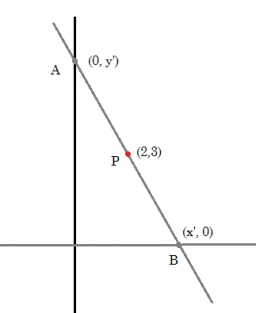# Intersection 8295

Write whether the function is ascending or descending and determine the coordinates of the intersection with the x and y axes:
y = 3x-2
y = 5x + 5
y = -0.5x-1

Result

r0 = (Correct answer is: R)x =  0.6667
y =  -2
r1 = (Correct answer is: R)u =  -1
v =  5
r2 = (Correct answer is: K)q =  -2
w =  -1
r3 = (Correct answer is: K)m =  2
n =  1

### Step-by-step explanation:

0 = 3·x-2

3x = 2

x = 2/3 = 0.66666667

x = 2/3 ≈ 0.666667

Our simple equation calculator calculates it.

y = 3·0-2

y = -2

y = -2/1 = -2

y = -2

Our simple equation calculator calculates it.

0 = 5·u+5

5u = -5

u = -5/5 = -1

u = -1

Our simple equation calculator calculates it.

v = 5·0+5

v = 5

v = 5/1 = 5

v = 5

Our simple equation calculator calculates it.

0 = -0.5·q-1

0.5q = -1

q = -1/0.5 = -2

q = -2

Our simple equation calculator calculates it.

w = -0.5·0-1

w = -1

w = -1/1 = -1

w = -1

Our simple equation calculator calculates it.

0 = -0.5·m+1

0.5m = 1

m = 1/0.5 = 2

m = 2

Our simple equation calculator calculates it.

n = -0.5·0+1

n = 1

n = 1/1 = 1

n = 1

Our simple equation calculator calculates it.Did you find an error or inaccuracy? Feel free to write us. Thank you!

Tips for related online calculators
Line slope calculator is helpful for basic calculations in analytic geometry. The coordinates of two points in the plane calculate slope, normal and parametric line equation(s), slope, directional angle, direction vector, the length of the segment, intersections of the coordinate axes, etc.
Do you have a linear equation or system of equations and looking for its solution? Or do you have a quadratic equation?